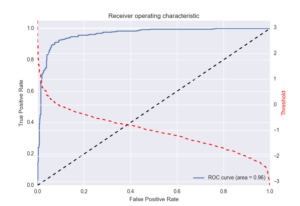Select Page

Several statistical and machine learning applications call for setting thresholds. For example, if you are presenting search results and wish to display short-listed results. Most document retrieval methods will generate a score per document and one way of showing the relevant documents is to set a threshold on the scores. Documents with scores below that threshold are not shown. Similarly, in anomaly detection, the system needs to alert whenever an anomaly is detected, or rather whenever the anomaly score exceeds a threshold.

While there are possibly many strategies for identifying and setting the best thresholds, the relationship of Receiver Operating Characteristics (ROC) to the thresholds provides an intuitive and flexible way to set thresholds. ROC is a curve of True Positive Rate (TPR) to the False Positive Rate (FPR) for decreasing values
of the score threshold. It can be computed in a few steps:

1. Sort the scores in a descending order
3. Compute the TPR and FPR for the current threshold and record it
4. Lower the threshold to the next unique score and go back to step 3 till all the scores have been exahausted

The formulae for computing TPR and FPR at a given score $latex s$ are quite simple:

$latex \text{TPR}_s = \frac{\text{Number of true positives with score above } s} {\text{Total number of positives}}$

$latex \text{FPR}_s = \frac{\text{Number of false positives with score above } s} {\text{Total number of negatives}}$

Or, quite simply, use the sklearn.metrics.roc_curve function from the scikit-learn package for computing ROC. That returns matched lists of TPR, FPR, and corresponding thresholds.

Once you have these three series (TPR, FPR, and thresholds), you just analyze the ROC curve to arrive at a suitable threshold. You plot the curve and identify the point along the ROC curve that is satisfactory to your needs (high TPR with low FPR). Since you will seldom find perfect TPR for zero FPR, you will have to make a compromise and allow for some false positives to cover most true positives. Choose a threshold that satifsfy the outcomes you are after.

Here’s code for generating and plotting the roc curve along with the corresponding thresholds

from sklearn.metrics import roc_curve, auc
import matplotlib.pyplot as plt
import numpy as np
import seaborn
from sklearn.datasets import make_classification

# sample data generation for demonstration only
x,y = make_classification(n_samples=10000, n_features=1, n_informative=1, n_redundant=0,n_repeated=0, n_clusters_per_class=1)
scores = x[:,0]
true_labels = y

### actual code for roc + threshold charts start here
# compute fpr, tpr, thresholds and roc_auc
fpr, tpr, thresholds = roc_curve(true_labels, scores)
roc_auc = auc(fpr, tpr) # compute area under the curve

plt.figure()
plt.plot(fpr, tpr, label='ROC curve (area = %0.2f)' % (roc_auc))
plt.plot([0, 1], [0, 1], 'k--')
plt.xlim([0.0, 1.0])
plt.ylim([0.0, 1.05])
plt.xlabel('False Positive Rate')
plt.ylabel('True Positive Rate')
plt.legend(loc="lower right")

# create the axis of thresholds (scores)
ax2 = plt.gca().twinx()
ax2.plot(fpr, thresholds, markeredgecolor='r',linestyle='dashed', color='r')
ax2.set_ylabel('Threshold',color='r')
ax2.set_ylim([thresholds[-1],thresholds])
ax2.set_xlim([fpr,fpr[-1]])

plt.savefig('roc_and_threshold.png')
plt.close()


A sample chart generated by this script is shown below. In the chart, the dashed black line is the baseline and your curve (the blue line) should be above that baseline, if your alogrithm is any good. In this case, we see that initially the TPR rises very fast, for a low FPR (that is a good thing) and later, the gains are not as significant. In general, a good first strategy is to choose the threshold that corresponds to the bend along the ROC curve. In this case, that corresponds roughly to a threshold of 0.